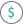#DOLLARFR (PQL - xl)

This function converts a decimal number dollar price to a fraction dollar price

• Library: PQL \ Spreadsheet \ Financial
• Compatibility: Any content (regardless of data source) in the Tabulate spreadsheet module

#### Syntax

DOLLARFR(decimal dollar, fraction)

##### Function Arguments
 Name Description Type Optional decimal dollar Decimal number Number fraction Denominator of the fraction; if not an integer, the number is truncated Number